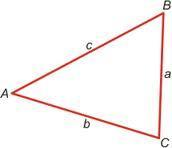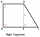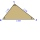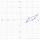# Triangle

The triangle has known all three sides: a=5.5 m, b=5.3 m, c= 7.8 m.
Calculate area of ​this triangle.

S =  14.5616 m2

### Step-by-step explanation:

Try calculation via our triangle calculator.Did you find an error or inaccuracy? Feel free to write us. Thank you!Tips to related online calculators

#### You need to know the following knowledge to solve this word math problem:

We encourage you to watch this tutorial video on this math problem:

## Related math problems and questions:

• Triangle SSSCalculate perimeter and area of ​​a triangle ABC, if a=53, b=46 and c=40.
• Triangle ABCCalculate the sides of triangle ABC with area 1404 cm2 and if a: b: c = 12:7:18
• TriangleCalculate heights of the triangle ABC if sides of the triangle are a=82, b=44, and c=53.
• Is right triangle or notIf right triangle ABC, have sides a=13, b=11.5, c=22.5. Find area.
• Calculate 7Calculate the height of the trapezoid ABCD, where coordinates of vertices are: A[2, 1], B[8, 5], C[5, 5] and D[2, 3]
• TentCalculate how many liters of air will fit in the tent that has a shield in the shape of an isosceles right triangle with legs r = 3 m long the height = 1.5 m and a side length d = 5 m.
• Sides ratioCalculate the circumference of a triangle with area 84 cm2 if a:b:c = 10:17:21
• Distributive propertyVerify the distributive property a×(b+c)=(a×b)+(a*c) for the rational number a=5/8, b=7/4 and c=2/3
• Heron backlawCalculate missing side in a triangle with sides 17 and 34 and area 275.
• Similarity coefficientThe ratio of similarity of two equilateral triangles is 3.5 (ie 7:2). The length of the side of smaller triangle is 2.4 cm. Calculate the perimeter and area of ​​the larger triangle.
• Area of RTCalculate the right triangle area that hypotenuse has length 14, and one hypotenuse segment has length 5.
• Similar trianglesTriangle A'B'C 'is similar to triangle ABC, whose sides are 5 cm, 8 cm, and 7 cm long. What is the length of the sides of the triangle A'B'C' if its circumference is 80 cm?
• Circles 2Calculate the area bounded by the circumscribed and inscribed circle in triangle with sides 12 cm, 14 cm, 18 cm.
• Is right-angledCan a triangle with the sides of sqrt 3, sqrt 5 and sqrt 8 (√3, √5 and √8) be a right triangle?
• Tangens parallelogramIf ∠BAD between the sides AB and AD of the parallelogram is θ, what is tan θ? See diagram: A=(7,1) B=(5,-2) C=(12,1) D=(14,4)
• TriangleDetermine whether we can make a triangle with the given side lengths. If so, use Heron's formula to find the area of the triangle. a = 158 b = 185 c = 201
• SquarePoints A[9,9] and B[-4,1] are adjacent vertices of the square ABCD. Calculate the area of the square ABCD.